# RFM Analysis Analysis Using Python

• Difficulty Level : Expert
• Last Updated : 08 Nov, 2021

In this article, we are going to see Recency, Frequency, Monetary value analysis using Python. But first, let us understand the RFM analysis briefly.

## What is RFM analysis?

RFM stands for recency, frequency, monetary value. In business analytics, we often use this concept to divide customers into different segments, like high-value customers, medium value customers or low-value customers, and similarly many others.

Attention geek! Strengthen your foundations with the Python Programming Foundation Course and learn the basics.

To begin with, your interview preparations Enhance your Data Structures concepts with the Python DS Course. And to begin with your Machine Learning Journey, join the Machine Learning - Basic Level Course

Let’s assume we are a company, our company name is geek, let’s perform the RFM analysis on our customers

1. Recency: How recently has the customer made a transaction with us
2. Frequency: How frequent is the customer in ordering/buying some product from us
3. Monetary: How much does the customer spend on purchasing products from us.

## Getting Started

Here we will import the required module( pandas, DateTime, and NumPy) and then read the data in the dataframe.

Dataset Used: rfm

## Python3

 `# importing necessary libraries``import` `pandas as pd``import` `datetime as dt``import` `numpy as np` `# importing the data``df ``=` `pd.read_excel( < my excel ``file` `location > )``df.head()`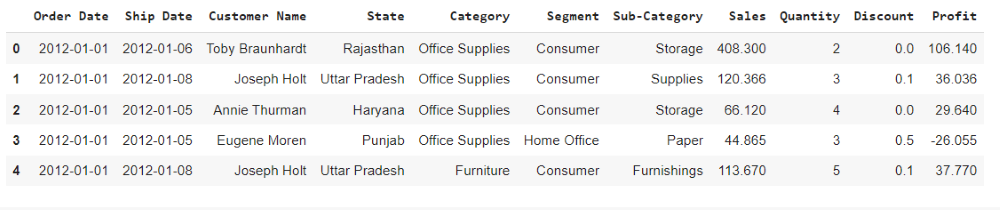### Calculating Recency

Here we are calculating recency for customers who had made a purchase with a company.

## Python3

 `df_recency ``=` `df.groupby(by``=``'Customer Name'``,``                        ``as_index``=``False``)[``'Order Date'``].``max``()``df_recency.columns ``=` `[``'CustomerName'``, ``'LastPurchaseDate'``]``recent_date ``=` `df_recency[``'LastPurchaseDate'``].``max``()``df_recency[``'Recency'``] ``=` `df_recency[``'LastPurchaseDate'``].``apply``(``    ``lambda` `x: (recent_date ``-` `x).days)``df_recency.head()`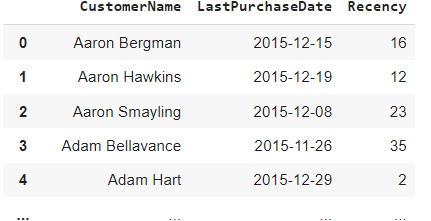### Calculating Frequency

We are here calculating the frequency of frequent transactions of the customer in ordering/buying some product from the company.

## Python3

 `frequency_df ``=` `df.drop_duplicates().groupby(``    ``by``=``[``'Customer Name'``], as_index``=``False``)[``'Order Date'``].count()``frequency_df.columns ``=` `[``'CustomerName'``, ``'Frequency'``]``frequency_df.head()`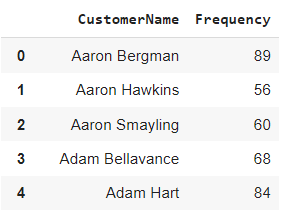### Calculating Monetary Value

Here we are calculating the monetary value of customer spend on purchasing products from the company.

## Python3

 `df[``'Total'``] ``=` `df[``'Sales'``]``*``df[``'Quantity'``]``monetary_df ``=` `df.groupby(by``=``'Customer Name'``, as_index``=``False``)[``'Total'``].``sum``()``monetary_df.columns ``=` `[``'CustomerName'``, ``'Monetary'``]``monetary_df.head()`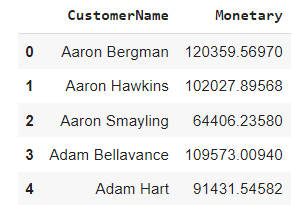### Merging all three columns in one dataframe

Here we are mering all the dataframe columns in a single entity using the merge function to display the recency, frequency, monetary value.

## Python3

 `rf_df ``=` `df_recency.merge(frequency_df, on``=``'CustomerName'``)``rfm_df ``=` `rf_df.merge(monetary_df, on``=``'CustomerName'``).drop(``    ``columns``=``'LastPurchaseDate'``)``rfm_df.head()`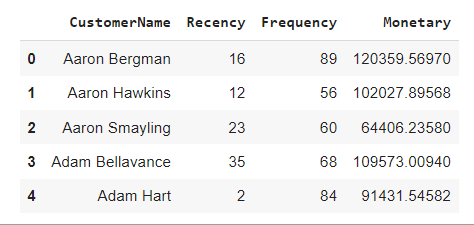### Ranking Customer’s based upon their recency, frequency, and monetary score

Here we are normalizing the rank of the customers within a company to analyze the ranking.

## Python3

 `rfm_df[``'R_rank'``] ``=` `rfm_df[``'Recency'``].rank(ascending``=``False``)``rfm_df[``'F_rank'``] ``=` `rfm_df[``'Frequency'``].rank(ascending``=``True``)``rfm_df[``'M_rank'``] ``=` `rfm_df[``'Monetary'``].rank(ascending``=``True``)` `# normalizing the rank of the customers``rfm_df[``'R_rank_norm'``] ``=` `(rfm_df[``'R_rank'``]``/``rfm_df[``'R_rank'``].``max``())``*``100``rfm_df[``'F_rank_norm'``] ``=` `(rfm_df[``'F_rank'``]``/``rfm_df[``'F_rank'``].``max``())``*``100``rfm_df[``'M_rank_norm'``] ``=` `(rfm_df[``'F_rank'``]``/``rfm_df[``'M_rank'``].``max``())``*``100` `rfm_df.drop(columns``=``[``'R_rank'``, ``'F_rank'``, ``'M_rank'``], inplace``=``True``)` `rfm_df.head()`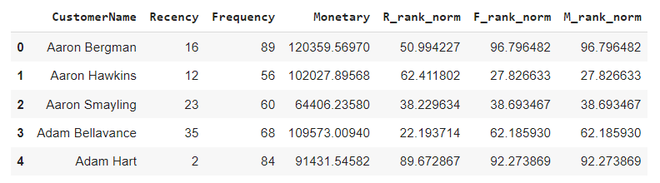### Calculating RFM score

RFM score is calculated based upon recency, frequency, monetary value normalize ranks. Based upon this score we divide our customers. Here we rate them on a scale of 5. Formula used for calculating rfm score is : 0.15*Recency score + 0.28*Frequency score + 0.57 *Monetary score

## Python3

 `rfm_df[``'RFM_Score'``] ``=` `0.15``*``rfm_df[``'R_rank_norm'``]``+``0.28` `*` `\``    ``rfm_df[``'F_rank_norm'``]``+``0.57``*``rfm_df[``'M_rank_norm'``]``rfm_df[``'RFM_Score'``] ``*``=` `0.05``rfm_df ``=` `rfm_df.``round``(``2``)``rfm_df[[``'CustomerName'``, ``'RFM_Score'``]].head(``7``)`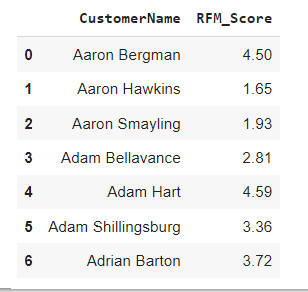### Rating Customer based upon the RFM score

• rfm score >4.5 : Top Customer
• 4.5 > rfm score > 4 : High Value Customer
• 4>rfm score >3 : Medium value customer
• 3>rfm score>1.6 : Low-value customer
• rfm score<1.6 :Lost Customer

## Python3

 `rfm_df[``"Customer_segment"``] ``=` `np.where(rfm_df[``'RFM_Score'``] >``                                      ``4.5``, ``"Top Customers"``,``                                      ``(np.where(``                                        ``rfm_df[``'RFM_Score'``] > ``4``,``                                        ``"High value Customer"``,``                                        ``(np.where(``    ``rfm_df[``'RFM_Score'``] > ``3``,``                             ``"Medium Value Customer"``,``                             ``np.where(rfm_df[``'RFM_Score'``] > ``1.6``,``                            ``'Low Value Customers'``, ``'Lost Customers'``))))))``rfm_df[[``'CustomerName'``, ``'RFM_Score'``, ``'Customer_segment'``]].head(``20``)`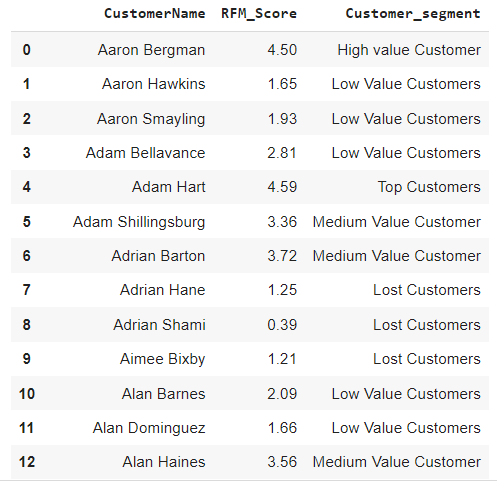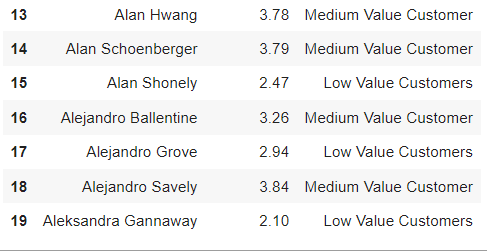### Visualizing the customer segments

Here we will use a pie plot to display all segments of customers.

## Python3

 `plt.pie(rfm_df.Customer_segment.value_counts(),``        ``labels``=``rfm_df.Customer_segment.value_counts().index,``        ``autopct``=``'%.0f%%'``)``plt.show()`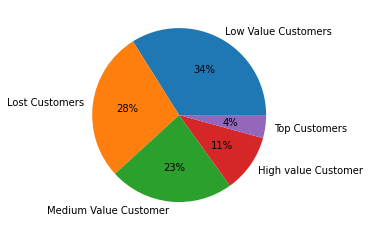My Personal Notes arrow_drop_up